# Simulation and Analysis of PI and NN Tuned PI Controllers for Transformer Based Three-Phase Multi-level Inverter with MC-PWM Techniques

Simulation and Analysis of PI and NN Tuned PI Controllers for Transformer Based Three-Phase Multi-level Inverter with MC-PWM Techniques
Department of Electrical and Electronics Engineering, Vignan’s Foundation for Science, Technology, and Research, Vadlamudi, Guntur 522213, India

Corresponding Author Email:
dmks_eeep@vignan.ac.in
Page:
587-598
|
DOI:
https://doi.org/10.18280/jesa.520606
25 June 2019
|
Accepted:
7 November 2019
|
Published:
30 December 2019
| Citation

OPEN ACCESS

Abstract:

The proposed work mainly deals with transformer-based three-phase Multi-Level Inverter (MLI) where the switches in MLI are controlled by Multi-Carrier PWM (MC-PWM) techniques. In this article, 48V DC input voltage is fed to 48/400V High-Voltage DC-DC boost converter which is controlled by Proportional–Integral (PI) and Neural-Network (NN) tuned PI controllers. 400V DC output from boost converter is connected to three-phase MLI where the MLI switches are controlled by three different types of MC-PWM techniques like Phase Disposition (PD), Phase Opposition and Disposition (POD) and Alternate Phase Opposition and Disposition (APOD). The overall system performance in terms of Total Harmonic Distortion (%THD) is analyzed and it is depicted that the proposed system under different modulation indices gives improved THD results with an NN tuned PI controller-based boost converter. The whole analysis is performed on the MATLAB\Simulink platform.

Keywords:

multi carrier PWM, multi-level inverter, PD, POD, APOD, THD

1. Introduction

Recently, many authors have reported their findings related to MLIs with SDC source instead of multiple sources for single and three-phase applications . A three-phase inverter has been investigated using an SDC input with single-phase transformers for grid-connected applications. Three phase MLIs using 3-phase transformers have been considered by the SDC source. This topology is suitable for grid-connected PV applications. All these topologies are used to enhance the output voltage level without increasing the number of levels, but, other hand utilization of transformers is also increased. From the discussion, it is found that all the inverter topologies are highly adaptable for standalone photovoltaic (PV) and grid connected applications . A three-phase topology has been designed with a reduced number of active switching devices, but it requires additional power diodes and DC bus capacitor. Recently, the inverter was designed for PV applications using the isolation transformer with three levels of the three-phase inverter . A three phase 4-wire PV interface grid-connected inverter was investigated. That generated a 3-level output voltage and required additional filtering circuits . For controlling an inverter, a different multi-carrier PWM technique has been applied .

For controlling the MLI different Multi-Carrier PWM (MC-PWM) techniques have been proposed, where for generating a five-level output voltage, M-1 carriers are considered. In the MC-PWM technique, M-1 number of carrier signals are compared with a reference signal. Based on the positioning of the carrier signals the PWM techniques are again classified as Alternate Phase Opposition Disposition (APOD), Phase Opposition Disposition (POD) and Phase Disposition (PD) . In this, a high-voltage DC-DC boost converter was used in front of MLI, for controlling the boost converter Proportional Integral (PI) and Neural Network (NN) tuned PI controllers are considered.

## 1.jpg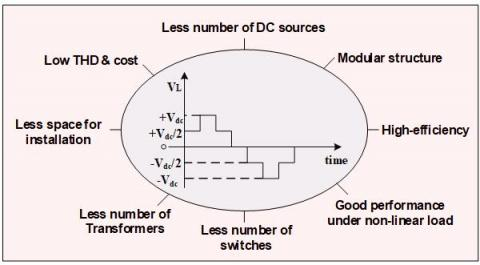Figure 1. Advantages of five-level inverter

The main objectives of the work are PI and NN tuned PI controllers are used to control the duty cycle of the boost converter similarly, for MLI controlling MC-PWM techniques are proposed . Then the overall performance of the system is observed in terms of Total Harmonic Distortion (%THD) analysis with the modulation index varying from 0.4 to 1. MLI has so many advantages that are clearly explained in Figure 1.

2. High-Voltage DC-DC Boost Converter

Isolation Sepic and fly back converter have come under Coupled Inductor (CI) DC-DC boost converters. The CI based converters produce high output voltage as compared to conventional boost converters. Regarding that, a CI-based High-voltage DC-DC converter was used for the three-phase MLI. The high-voltage dc/dc converter contains input dc voltage Vin, coupled inductor (CI), main switch S, one output capacitor C0, one output diode D0, one clamp capacitor C1, clamp diode D1, two diodes D2 and D3 and two capacitors C2 and C3. The CI contains leakage inductor (LI) Lk, magnetizing inductor (MI) Lm and an ideal transformer. Figure 2 represents the high-voltage DC-DC converter. PI and NN tuned PI controllers are applied to the boost converter for controlled the duty cycle. In this, a 48V DC voltage is given to the input of the boost converter and it produces 400V DC voltage at the output side . The operating stages of High-Voltage DC-DC converter is explained in Figure 3.

## 2.jpgFigure 2. High-voltage DC-DC converter

## 3.jpg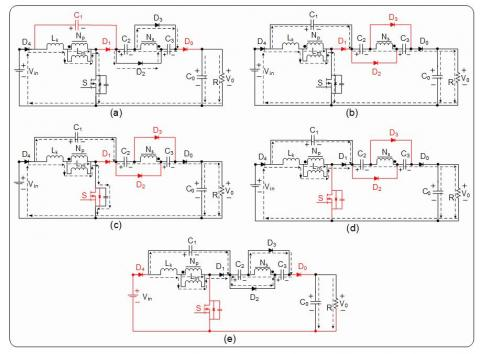Figure 3. Stages of operation of the High-Voltage DC-DC converter

In stage one diodes D0 and D1 both are in off state remaining diodes and switch are in on state. In this case Vin supplies the energy to the Lk and Lm, due to Lk secondary side of the CI current is decreases linearly. So, load (R) receives the energy from Co only. In stage two diode Do and switch both are in on state remaining are in off state. In this case Vin supplies the energy to the Lk, Lm and secondary side of the CI. The capacitors C1, C2 and C3 are in series the voltage is transfer to both Co and Load (R). In stage three diode Do and switch both are in on state remaining are in off state. In this case Vin supplies the energy to the parasitic capacitor (Cds) of the main switch through the Lk, Lm. The capacitors C1, C2 and C3 are still in series the voltage is transform to both Co as well as Load (R). In stage four diodes D2 and 3 are in on state remaining diodes and switch are in off state. Input voltage source (Vin) supplies energy to the C1 through the Lk and Lm. Load (R) and capacitor Co gets energy from the secondary side of the CI. In stage five diode Do and switch both are in off state remaining diodes are in on state. The energy in the Lk and Lm are transfer to the C1 similarly, C3 and C3 are charged by Lk and Lm in the secondary side of the CI. Load (R) gets energy from Co only.

2.1 Modelling of the high-voltage DC-DC converter

Let, the energy in the Lk is transfer to the capacitor. Expression of the duty cycle Dc1 is

$D_{C 1}=\frac{t_{C 1}}{T_{S}}=\frac{2(1-D)}{n+1}$          (1)

where, tc1 =Time interval

Stages I and III are knowingly small, the remaining three Stages are taken in CMS analysis. In Stage II, based on Figure 3 (Stage 2).

$V_{L k}^{2}=\frac{L_{k 1}}{L_{m}+L_{k 1}} V_{m}=(1-k) V_{in}$     (2)

$V_{L 1}^{2}=\frac{L_{m}}{L_{m}+L_{k 1}} V_{i n}=k V_{in}$       (3)

$V_{L 2}^{2}=n V_{L 1}^{2}=n k V_{i n}$        (4)

$V_{0}=V_{i n}+V_{C 1}+V_{C 2}+V_{L 2}^{2}+V_{C 3}$       (5)

The subsequent equations are set, by utilizing the voltage second balance principle

$\int_{0}^{D T_{S}} V_{L k}^{2} d t+\int_{D T_{S}}^{T_{S}} V_{L k}^{5} d t=0$   (6)

$\int_{0}^{D T_{s}} V_{L 1}^{2} d t+\int_{D T_{S}}^{T_{S}} V_{L 1}^{5} d t=0$      (7)

$\int_{0}^{D T_{S}} V_{L 2}^{2} d t+\int_{D T_{S}}^{T_{S}} V_{L 2}^{5} d t=0$     (8)

Stage V voltage can be derived by substituting Eqns. (1), (2), (3) and (4) into Eqns. (6), (7) and (8). The voltages are expressed, according to the definition of voltage direction.

$V_{L k}^{5}=-\frac{D(n+1)(1-k)}{2(1-D)} V_{in}$     (9)

$V_{L 1}^{5}=-\frac{D k}{1-D} V_{i n}$          (10)

$V_{L 2}^{5}=-\frac{n D k}{1-D} V_{i n}$        (11)

In Stage V, capacitors $\mathrm{C}_{1}, \mathrm{C}_{2}$ and $\mathrm{C}_{3}$ also charged. Based on Figure 3 (Stage 5) capacitor voltage can be denoted as.

$V_{C 1}=-V_{L k}^{5}-V_{L 1}^{5}$$=\frac{D}{1-D} V_{i n} \frac{(1+k)+(1-k) n}{2}$          (12)

$V_{C 2}=V_{C 3}=-V_{L 2}^{5}=\frac{n D k}{1-D} V_{i n}$     (13)

To obtain voltage gain by substituting equations 4, 12 and 13 into equation 5.

$M_{C C M}=\frac{V_{0}}{V_{in}}=\frac{1+n k}{1-D}+\frac{D}{1-D} \frac{(k-1)+n(1+k)}{2}$    (14)

To obtain ideal voltage gain, at k=1

$M_{C C M}=\frac{1+n+n D}{1-D}$     (15)

Voltage stresses on diodes $\mathrm{D}_{0}, \mathrm{D}_{1}, \mathrm{D}_{2}$ and $\mathrm{D}_{3}$ and switch are specified as

$V_{d s}=\frac{1}{1-D} V_{in}=\frac{V_{0}+n V_{in}}{2 n+1}$       (16)

$V_{D 1}=\frac{1}{1-D} V_{in}=\frac{V_{0}+n V_{in}}{2 n+1}$      (17)

$V_{D 2}=V_{D 3}=V_{D 0}=\frac{n}{1-D} V_{i n}=\frac{n}{2 n+1}\left(V_{0}+n V_{in}\right)$   (18)

Eq. (16), (17) and (18) are in equal voltage ratio, by adjusting CI turns ratio the voltage stresses can be regulated.

3. Transformer-Based Three-Phase Multi-Level Inverter

MLIs are mostly used in grid-connected and various AC drive applications . The fundamental topologies for MLIs are Cascaded H-Bridge, Flying-capacitors, and Diode-clamped. All these fundamental topologies have their own merits and demerits, the fundamental topologies need a slightly huge number of switch count and occupy huge space. So, a new MLI topology was presented in this article.

The projected MLI topology has a very less number of switches as related to existing topologies and it produces minimum switching losses and probably less %THD. The projected topology is operated in five modes. Figure 4 indicates the block diagram of the transformer-based three-phase multi-level inverter. MLI contains two capacitors, four diodes, and five switches. The switch 5 contains four diodes which are arranged as a bridge where switch 1 and 2 are in one leg and switch 3 and 4 are in another leg. Out of five switches, switch 1, 2 and 5 function at high frequency and the remaining two switches function at low frequency. Here low switching frequency and high switching frequency are referred to as fundamental frequency and carrier signal frequency respectively. Figure 5 indicates the four modes of operation of the inverter. In stage one, switches Sa4 and Sa5 are active and the remaining switches Sa1, Sa2 and Sa3 are inactive which results in the output voltage +V0/2. In stage two, only switches Sa1 and Sa4 are active, results+V0. In the third stage, only switches Sa3 and Sa5 are active, which gives output voltage-V0/2. In stage four, only switches Sa2 and Sa3 are active, results -V0 as output voltage. Finally, a five-level output voltage is obtained only by using five switches. Table 1 depicts the switching modes of five-level MLI. Figure 6 represents the inverter voltages and currents at various modulation index values.

## 4.jpg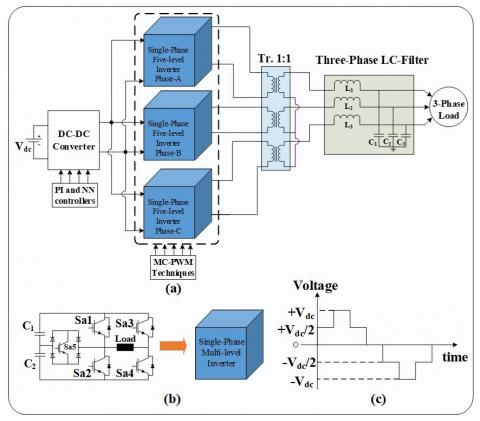Figure 4. Block diagram of the transformer-based three-phase MLI

## 5.jpg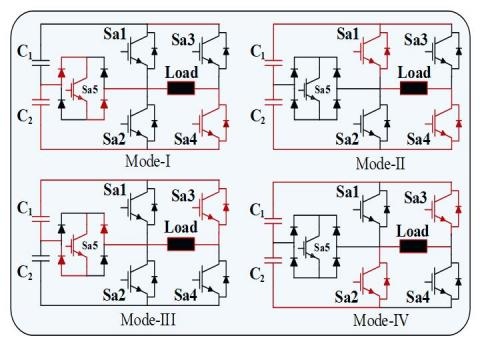Figure 5. Four stages of operation of the inverter

Table 1. Switching modes of the inverter

 S. No S1 S2 S3 S4 S5 Voltage (V0) 1 X X X √ √ +V0/2 2 √ X X √ X +V0 3 √(or)X X(or)√ √(or)X X(or)√ X 0 4 X X √ X √ -V0/2 5 X √ √ X X +V0

## 6.png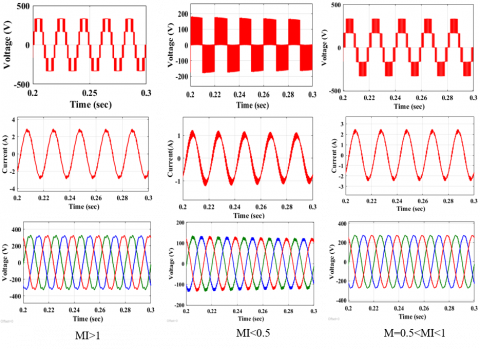Figure 6. Inverter output voltages and currents with different MI values

Table 2 indicates the comparison among the existing MLI topologies and suggested MLI topology which represents that suggested topology has less number of switches compared to the existing topologies [15, 16].

Table 2. Comparison of components utilized in between the existing topologies and suggested topology

 Reference Components utilization Active Switches Input DC Sources DC Bus Capacitors Transformers Total [R-14] 24 01 01 06 32 [R-15] 24 01 01 02 28 [R-16] 18 03 03 01 25 Suggested 15 01 02 01 19
4. Control Techniques

4.1 Multi-carrier pulse width modulation

In the MC-PWM techniques, four triangles are compared with a single sine wave to produce a pulse, in this M-1 triangles are considered for an M-level inverter. Here, four triangles are considered for an MC-PWM technique all triangles have equal magnitude and frequency. The Modulation Index (MI) of the inverter is given in (1)

$M_{inv}=\frac{A_{m}}{(m-1) A_{C}}$       (19)

where, Am represents the amplitude of the modulation signal and Ac represents the amplitude of the carrier signal. When Am>Ac then only produces a positive pulse remaining condition no pulse is produced. The MC-PWM techniques are categorized into three types such as Phase Disposition (PD), Phase Opposite Disposition (POD) and Alternative Phase Opposite Disposition (APOD). In this, carrier waves contain 2k Hz frequency and sine wave contains 50 Hz frequency. Hence, the Frequency Modulation ratio is $\mathrm{Mf}=\left(f_{s} / f_{r}\right)$. Each carrier is compared with a sine wave to produce pulses for MLI switches. The switching logic of the PD is presented in Figure 7. The similar way POD and APOD are also derived using logic gates.

## 7.jpgFigure 7. Switching logic

## 8.jpg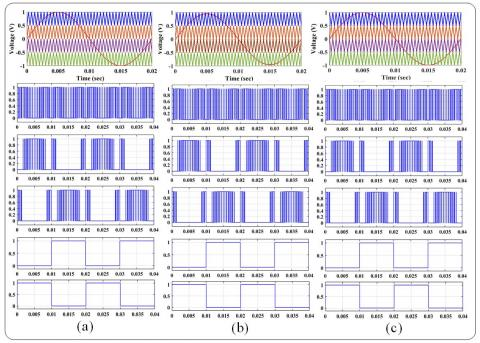Figure 8. Multicarrier PWM techniques (a) PD (b) POD (c) APOD

In PD-PWM technique four carrier signals are used for generating switching pulses, all four carrier signals having equal amplitude and in phase with each other and the switching pattern also given in Figure 8 (a). In the POD-PWM technique, four carrier signals are used for generating switching pulses, out of four carrier signals, two carrier waves are in-phase with each other with above zero level and the other two carrier signals are maintained 1800 phase shift with below the zero points. All carrier signals are maintained the equal amplitude and frequency, but the difference in phase shift and the switching pattern also given in Figure 8 (b). In APOD-PWM, zero reference is placed in the middle of the carriers. Above the zero references, carriers are positive and below the zero references are negative carriers. In this, all carriers are maintaining 1800 shifted with each other and the switching pattern of AOPD is given in Figure 8 (c).

4.2 Proportional integral

The PI controller contains mainly two parameters those are Proportional and Integral. The transfer function of the PI controller is as follows:

$G_{C}(s)=K_{p}\left(1+\frac{1}{K_{i} S}\right)=K_{p}\left(\frac{K_{i} S+1}{K_{i} S}\right)$     (20)

where, Kp indicates the proportional constant and Ki indicates the integral constant. Figure 9 indicates the block diagram of the PI controller with unity feedback

## 9.jpg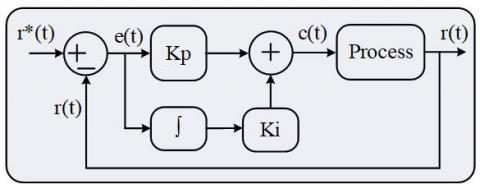Figure 9. Block diagram of the PI controller

From Figure 9 r*(t) indicates the reference value, r(t) indicates the actual value and e(t) indicates the error value. Most of the researchers prefer a PI controller for easier operation and implementation. The main purpose of the PI controller is to give fast response, to regulates the peak undershoot, settling time and peak overshoot of the system.

4.3 Neural network controller

NN algorithms are inspired by the structure of the human brain. NN takes data themselves and self-train to get the desired output. NN is made up of several layers of neurons these neurons are core processing units of the network. NN controller has mainly three layers those are input, output, and hidden layers. The first input layer will seed the input, the output layer predicts the final output in between the existing hidden layers. Neurons of one layer are connected to the neurons of the next layer through the channels. Each of these channels is assigned a numerical value known as weights. From Figure 10 it is clearly observed that X1, X2 and X3 represents the input values, Y1 and Y2 represents the output values and W1 represents the weights of the network. Figure 10 indicates the neural network architecture.

## 10.jpg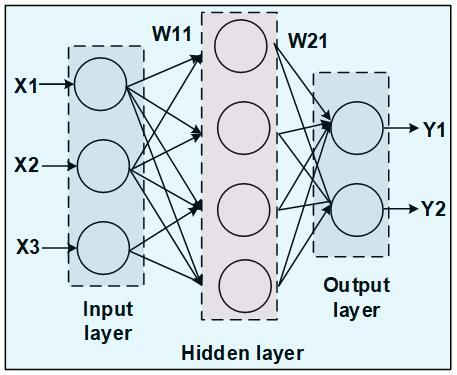Figure 10. The architecture of the neural network

In case if the output is not satisfied with the requirement by adjusting the weights of the network desired accurate output can be found. In the NN controller, so many learning algorithms are available those are supervised learning, Incremental, and Batch training . Out of all these algorithms, an Incremental algorithm is stated as an ‘adaptive’ or ‘online’ training algorithm. The incremental algorithm is easily adaptable to the unknown situation, it can solve complex functions, easy to learn and train. Finally, it produces a better response of the system, with less settled time and a smooth response.

## 11.jpg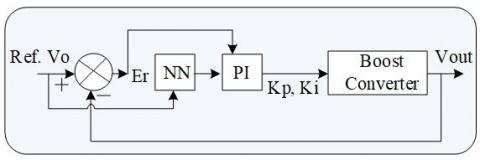Figure 11. Closed loop control of the high-voltage DC-DC converter

The simplified block diagram of NN tuned PI controller for High-voltage DC-DC converter to get the required output voltage is shown in the Figure 11. The actual output voltage is compared to the reference output voltage Vref. The error signal Er is obtained and is applied to the Neural Network tuned PI controller or PI controller. The output signal from the controller is applied to the power switch as gating signal. The neural network is trained with the best kp and ki values of the PI controller for various reference output voltages of High-Voltage DC-DC converter.

5. Results

PI and NN tuned PI control techniques are applied to the high-voltage DC-DC boost converter and MC-PWM control techniques are applied to the transformer-based three-phase multi-level inverter. Figure 12 represents DC-DC boost converter output voltage and, Figure 13, 14 and 15 represent the inverter voltages and currents with PI and NN tuned PI control techniques respectively.

Figure 12 (a) is illustrating the PI-based boost converter output voltage, it is settled at 0.15sec. Similarly, Figure 12 (b) is illustrating the NN tuned PI controller-based boost converter output voltage is settled at 0.07sec. Settling time in case of NN tuned PI controller is less when compared to the PI controller along with less ripples and less overshoot with the NN tuned PI controller.

Figure 13 illustrate the PI-based inverter output voltages, it is settled at 0.065sec. Similarly, the NN tuned PI controller-based inverter output voltages are settled at 0.045sec. Settling time in case of NN tuned PI controller is less when compared to the PI controller along with less ripples and less overshoot with the NN tuned PI controller.

Figure 14 is illustrating the PI-based inverter output currents, it is settled at 0.067sec. Similarly, the NN tuned PI-based inverter output currents are settled at 0.047sec. Settling time in case of NN tuned PI controller is less when compared to the PI controller along with less ripples and less overshoot with the NN tuned PI controller.

## 12.png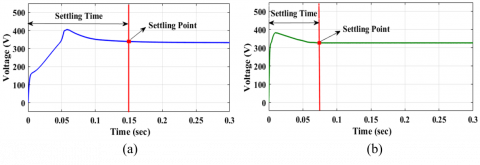Figure 12. Boost converter output voltages (a) with PI controller (b) with NN tunes PI controller

## 13.pngFigure 13. Inverter output voltages (a) PI-PD (b) PI-POD (c) PI-APOD (d) NN tuned PI-PD (e) NN tuned PI-POD (f) NN tuned PI-APOD

## 14.png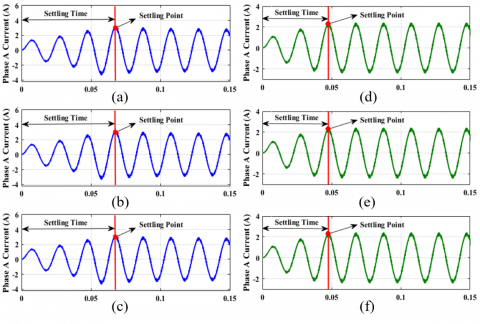Figure 14. Inverter output currents (a) PI-PD (b) PI-POD (c) PI-APOD (d) NN tuned PI-PD (e) NN tuned PI-POD (f) NN tuned PI-APOD

## 15.png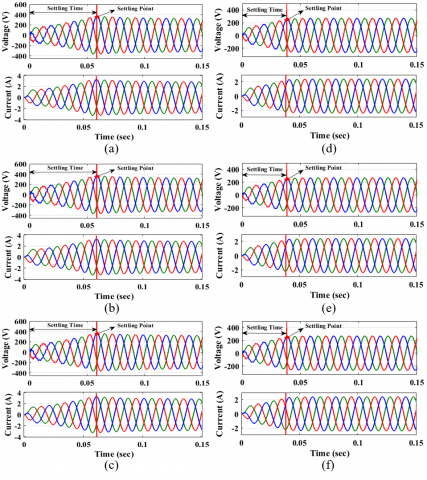Figure 15. Three-phase inverter output voltage and current (a) PI-PD (b) PI-POD (c) PI-APOD (d) NN tuned PI-PD (e) NN tuned PI-POD (f) NN tuned PI-APOD

Figure 15 is illustrating the PI-based three-phase inverter output voltages and currents. it is settled at 0.067sec. Similarly, the NN tuned PI-based three-phase inverter output voltages and currents are settled at 0.038sec. Settling time in case of NN tuned PI controller is less when compared to the PI controller along with less ripples and less overshoot with the NN tuned PI controller.

The settling time and settling point of the inverter output waveforms are represented in the Table 3. The settling time and settling point in case of NN tuned PI controller is less when compared to PI controller

Table 4 represents the THD analysis of the PD technique to MLI with PI and NN tuned PI controllers, to boost converter, by varying the modulation index from 0.4 to 1. The PI controller-based system has produced 2.43% THD while the NN tuned PI controller-based system has produced 2.21% THD at (Modulation Index) MI=1. Finally, the NN tuned PI controlled based system has produced less THD as compared to the PI controller.

Table 5 represents the THD analysis of the POD technique to MLI with PI and NN tuned PI controllers, to boost converter, by varying the modulation index from 0.4 to 1. The PI controller-based system has produced 2.44% THD while the NN tuned PI controller-based system has produced 2.22% THD at (Modulation Index) MI=1. Finally, the NN tuned PI controlled based system has produced less THD as compared to the PI controller.

Table 6 represents the THD analysis of the APOD technique to MLI with PI and NN tuned PI controllers, to boost converter, by varying the modulation index from 0.4 to 1. The PI controller-based system has produced 2.48% THD while the NN tuned PI controller-based system has produced 2.26% THD at (Modulation Index) MI=1. Finally, the NN tuned PI controlled based system has produced less THD as compared to the PI controller.

Figure 16 represents the THD analysis of the MLI system. Figure 16 (a) indicates the THD of PD-MLI with PI controller has produces 2.43% THD, Figure 16 (b) indicates the THD of POD-MLI with PI controller has produces 2.44% THD, Figure 16 (c) indicates THD of APOD-MLI with PI controller has produces 2.48% THD. Similarly, Figure 16 (d) indicates THD of PD-MLI with NN tuned PI controller has produces 2.21% THD, Figure 16 (e) indicates THD of POD-MLI with NN tuned PI controller has produces 2.22% THD finally Figure 16 (f) indicates THD of APOD-MLI with NN tuned PI controller has produces 2.26% THD.

Table 7 represents the THD analysis of the MLI system with PI and NN tuned PI controllers. From the Table 7 to clearly observe the PD-PWM technique with the NN tuned PI controller significantly improve the performances of the inverter as compared to the other techniques and current THD is also within the IEEE standards.

Table 3. Summary of PI and NN tuned PI controllers

 Parameters PI Controller NN tuned PI Controller Settling time Settling point Settling time Settling point DC output voltage 0.0 to 0.15 0.15 0.0 to 0.07 0.07 Inverter  voltages 0.0 to 0.065 0.065 0.0 to 0.045 0.045 Inverter currents 0.0 to 0.067 0.067 0.0 to 0.047 0.047 Three-phase output 0.0 to 0.067 0.058 0.0 to 0.038 0.038

Table 4. Summary of phase disposition

 Modulation index MLI with  PI controller MLI with NN tuned PI controller Voltage (Vph) % THD Current (A) % THD Voltage (Vph) % THD Current (A) % THD 1 215.3 15.74 1.87 2.43 215.6 15.70 1.87 2.21 0.9 194.9 16 1.69 2.46 195.1 15.91 1.69 2.20 0.8 175 20.2 1.51 2.65 175.1 19.94 1.52 2.46 0.7 154.5 22.33 1.33 2.88 154.8 22.25 1.34 2.61 0.6 133.1 23.47 1.14 3.18 132.8 23.34 1.13 2.71 0.5 114.3 32.62 0.95 3.86 114.0 32.45 0.95 2.79 0.4 94.96 38.59 0.78 3.14 94.89 38.49 0.77 2.91

Table 5. Summary of phase opposite disposition

 Modulation index MLI with PI controller MLI with NN tuned PI controller Voltage (Vph) % THD Current (A) % THD Voltage (Vph) % THD Current (A) % THD 1 216.8 19.87 1.87 2.44 216.8 19.80 1.88 2.22 0.9 199.6 27.46 1.69 2.57 199.7 27.42 1.69 2.32 0.8 180.7 32.71 1.51 2.79 180.8 32.68 1.52 2.60 0.7 160.2 35.66 1.33 3.02 160.0 35.62 1.34 2.77 0.6 137.5 35.33 1.14 3.28 137.5 35.25 1.13 2.84 0.5 115.9 36.84 0.95 3.88 115.8 36.67 0.94 2.82 0.4 104.1 61.81 0.78 3.56 104.0 61.73 0.78 3.36

Table 6. Summary of alternate phase opposite disposition

 Modulation index MLI with PI controller MLI with NN tuned PI controller Voltage (Vph) % THD Current (A) % THD Voltage (Vph) % THD Current (A) % THD 1 218.5 23.42 1.87 2.48 217.6 23.35 1.87 2.26 0.9 199.0 26.27 1.69 2.56 199.2 26.22 1.69 2.31 0.8 177.9 27.27 1.51 2.71 177.9 27.22 1.51 2.52 0.7 155.8 25.84 1.33 2.88 160.1 25.78 1.34 2.62 0.6 133.2 23.60 1.14 3.16 133.1 23.49 1.13 2.69 0.5 115.9 36.84 0.95 3.88 115.2 36.67 0.94 2.82 0.4 104.1 61.81 0.78 3.56 104.0 61.73 0.78 3.36

Table 7. Summary of % THD with PI and NN tuned PI controllers

 Modulation Technique % THD with PI Controller % THD with NN tuned PI Controller PD 2.43 2.21 POD 2.44 2.22 APOD 2.48 2.26

## 16.png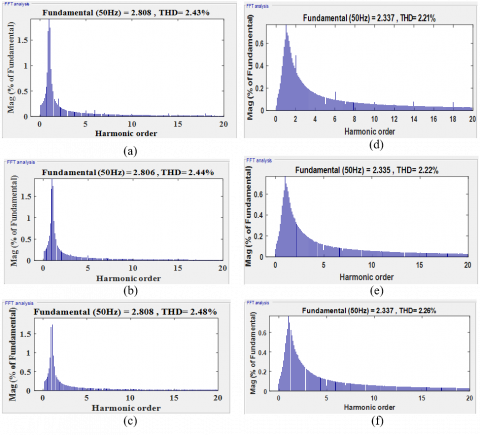Figure 16. THD analysis of the MLI with PI and NN tuned PI controllers

6. Conclusion

This article mainly discussed a transformer-based three-phase multi-level inverter with MC-PWM techniques that comprises a minimum number of switches which is peculiar than existing topologies [13-15]. This MLI topology is simulated using only 15 power semiconductor switches and it produces minimum switching losses. Multi-carrier PWM techniques like PD, POD, and APOD are applied to control the MLI switches. The inverter topology is given an input supply of 400V AC which is derived from the high-voltage DC-DC boost converter. The boost converter operates at 48V DC input voltage source and it is boosted to the general operating voltage of 400V DC. In that DC-DC boost converter is controlled by two different controllers namely PI and NN tuned PI controllers. Comparative analysis in terms of THD in MLI's output voltage and output current is done on PI-based DC-DC boost converter and NN tuned PI based DC-DC boost converter. From Table 7 it can be depicted that NN tuned PI based DC-DC boost converter with MLI resulted in less THD than PI-based DC-DC boost converter with MLI at different modulation indices. Similarly, settling time in case of the NN tuned PI controller is less when compared to the PI controller along with less ripples and less overshoot with the NN tuned PI controller.

Acknowledgment

The authors are very thankful to the management of Vignan’s Foundation for Science, Technology, & Research for the successful completion of the work.

Nomenclature
 MLI Multilevel Inverter MC-PWM Multi Carrier Pulse Width Modulation PD Phase Disposition POD Phase opposite Disposition APOD Alternate Phase opposite Disposition PI proportional–integral NN Neural Network MI Modulation Index
References

 Gupta, K.K., Jain, S. (2014). A novel multilevel inverter based on switched DC sources. IEEE Transactions on Industrial Electronics, 61(7): 3269-3278. http://dx.doi.org/10.1109/TIE.2013.2282606

 Kavali, J., Mittal, A. (2016). Analysis of various control schemes for minimal total harmonic distortion in cascaded H-bridge multilevel inverter. Journal of Electrical Systems and Information Technology, 3: 428-441. http://dx.doi.org/10.1016/j.jesit.2016.01.007

 Ali, J.S.M., Krishnaswamy, V. (2018). An assessment of recent multilevel inverter topologies with reduced power electronics components for renewable applications. Renewable and Sustainable Energy Reviews, 82(3): 3379-3399. https://doi.org/10.1016/j.rser.2017.10.052

 Pires, V.F., Cordeiro, A., Foito, D., Silva, J.F. (2018). Three-phase multilevel inverter for grid-connected distributed photovoltaic systems based in three three-phase two-level inverters. Solar Energy, 174: 1026-1034. https://doi.org/10.1016/j.solener.2018.09.083

 Jana, J., Saha, H., Bhattacharya, K.D. (2017). A review of inverter topologies for single-phase grid-connected photovoltaic systems. Renewable and Sustainable Energy Reviews, 72: 1256-1270. https://doi.org/10.1016/j.rser.2016.10.049

 Kala, P., Arora, S. (2017). A comprehensive study of classical and hybrid multilevel inverter topologies for renewable energy applications. Renewable and Sustainable Energy Reviews, 76: 905-931. https://doi.org/10.1016/j.rser.2017.02.008

 Prabaharan, N., Palanisamy, K. (2017). A comprehensive review on reduced switch multilevel inverter topologies, modulation techniques and applications. Renewable and Sustainable Energy Reviews, 76: 1248-1282. https://doi.org/10.1016/j.rser.2017.03.121

 Ramya, G., Ganapathy, V., Suresh, P. (2019). Comprehensive analysis of interleaved boost converter with simplified H-bridge multilevel inverter based static synchronous compensator system. Electric Power Systems Research, 176(105936): 1-14. https://doi.org/10.1016/j.epsr.2019.105936

 Suresh, Y., Panda, A.K. (2013). Investigation on hybrid cascaded multilevel inverter with reduced dc sources. Renewable and Sustainable Energy Reviews, 26: 49-59. https://doi.org/10.1016/j.rser.2013.04.027

 Colak, I., Kabalci, E., Bayindir, R. (2011). Review of multilevel voltage source inverter topologies and control schemes. Energy Conversion and Management, 52(2): 1114-1128. https://doi.org/10.1016/j.enconman.2010.09.006

 Venkatesan, M., Rajeswari, R., Deverajan, N. (2015). A fuzzy logic based three phase inverter with single DC source for grid connected PV system employing three phase transformer. International Journal of Renewable Energy Research, 5(3): 739-745. https://doi.org/10.3906/elk-1404-423

 Susheela, N., Kumar, P.S. (2017). Performance evaluation of carrier based PWM techniques for hybrid multilevel inverters with reduced number of components. Energy Procedia, 117: 635-642. https://doi.org/10.1016/j.egypro.2017.05.164

 Hsieh, Y.P., Chen, J.F., Liang, T.J., Yang, L.S. (2013). Novel high step-up DC–DC converter for distributed generation system. IEEE Transactions on Industrial Electronics, 60(4): 1473-1482. http://dx.doi.org/10.1109/TIE.2011.2107721

 Singh, J., Dahiya, R., Saini, L.M. (2018). Recent research on transformer based single DC source multilevel inverter: A review. Renewable and Sustainable Energy Reviews, Renewable, 82(3): 3207-3224. https://doi.org/10.1016/j.rser.2017.10.023

 Song, S.G., Kang, F.S., Park, S.J. (2019). Cascaded multilevel inverter employing three-phase transformers and single DC input. IEEE Transactions on Industrial Electronics, 56(6): 2005-2014. http://dx.doi.org/10.1109/TIE.2009.2013846

 Pires, V.F., Cordeiro, A., Foito, D., Silva, F. (2018). Three-phase multilevel inverter for grid-connected distributed photovoltaic systems based in three three-phase two-level inverters. Solar Energy, 174: 1026-1034. https://doi.org/10.1016/j.solener.2018.09.083

 Fu, X., Li, S., Fairbank, M., Wunsch, D.C., Alonso, E. (2015). Training recurrent neural networks with the levenberg–marquardt algorithm for optimal control of a grid-connected converter. IEEE Transactions on Neural Networks and Learning Systems, 26(9): 1900-1912. http://dx.doi.org/10.1109/TNNLS.2014.2361267